NABARD Quantitative Aptitude Practice: 3

# NABARD Quantitative Aptitude Practice: 3

Test Description

## 30 Questions MCQ Test NABARD Assistant Manager Grade B Mock Test Series | NABARD Quantitative Aptitude Practice: 3

NABARD Quantitative Aptitude Practice: 3 for Banking Exams 2022 is part of NABARD Assistant Manager Grade B Mock Test Series preparation. The NABARD Quantitative Aptitude Practice: 3 questions and answers have been prepared according to the Banking Exams exam syllabus.The NABARD Quantitative Aptitude Practice: 3 MCQs are made for Banking Exams 2022 Exam. Find important definitions, questions, notes, meanings, examples, exercises, MCQs and online tests for NABARD Quantitative Aptitude Practice: 3 below.
Solutions of NABARD Quantitative Aptitude Practice: 3 questions in English are available as part of our NABARD Assistant Manager Grade B Mock Test Series for Banking Exams & NABARD Quantitative Aptitude Practice: 3 solutions in Hindi for NABARD Assistant Manager Grade B Mock Test Series course. Download more important topics, notes, lectures and mock test series for Banking Exams Exam by signing up for free. Attempt NABARD Quantitative Aptitude Practice: 3 | 30 questions in 25 minutes | Mock test for Banking Exams preparation | Free important questions MCQ to study NABARD Assistant Manager Grade B Mock Test Series for Banking Exams Exam | Download free PDF with solutions
 1 Crore+ students have signed up on EduRev. Have you?
NABARD Quantitative Aptitude Practice: 3 - Question 1

### Three Science classes A, B and C take a Life Science test. The average score of class A is 83. The average score of class B is 76. The average score of class C is 85. The average score of class A and B is 79 and average score of class B and C is 81. Then the average score. Of classes A, B and C is

Detailed Solution for NABARD Quantitative Aptitude Practice: 3 - Question 1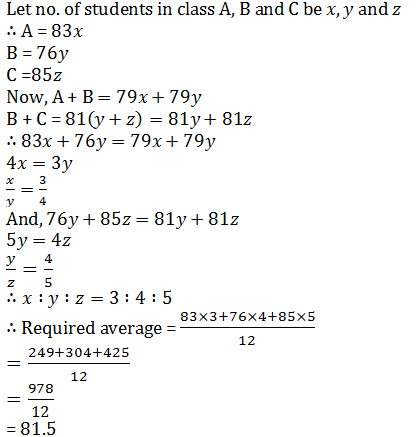NABARD Quantitative Aptitude Practice: 3 - Question 2

### There are 50 students in a class. Their average weight is 45 kg. When one student leaves the class the average weight reduces by 100g. What is the weight of the student who left the class?

Detailed Solution for NABARD Quantitative Aptitude Practice: 3 - Question 2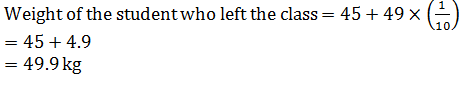NABARD Quantitative Aptitude Practice: 3 - Question 3

### The simple interest on a sum of money at 4% per annum for 2 years is Rs. 80. The compound interest in the same for the same period is :

Detailed Solution for NABARD Quantitative Aptitude Practice: 3 - Question 3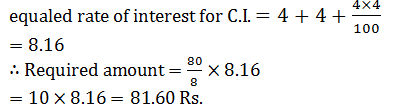NABARD Quantitative Aptitude Practice: 3 - Question 4

200 litres of a mixture contains 15% water and the rest is milk. The amount of milk that must be added so that the resulting mixture contains 87.5% milk is

Detailed Solution for NABARD Quantitative Aptitude Practice: 3 - Question 4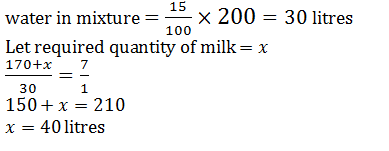NABARD Quantitative Aptitude Practice: 3 - Question 5

In an examination, 60% of the candidates passed in English and 70% of the candidates passed in Mathematics, but 20% failed in both of these subjects. If 2500 candidates passed in both the subjects the number of candidates who appeared at the examination was :

Detailed Solution for NABARD Quantitative Aptitude Practice: 3 - Question 5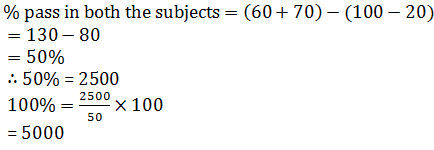NABARD Quantitative Aptitude Practice: 3 - Question 6

Ramesh bought 10 cycles for Rs. 500 each. He spent Rs. 2,000 on the repair of all cycles, He sold five of them for Rs. 750 each and the remaining for Rs. 550 each. Then the total gain or loss % is

Detailed Solution for NABARD Quantitative Aptitude Practice: 3 - Question 6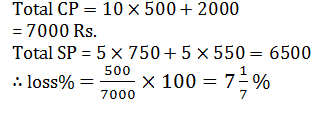NABARD Quantitative Aptitude Practice: 3 - Question 7

The milk and water in a mixture are in the ratio 7 : 5. When 15 litres of water are added to it, the ratio of milk and water in the new mixture becomes 7 : 8. The total quantity of water in the new mixture is

Detailed Solution for NABARD Quantitative Aptitude Practice: 3 - Question 7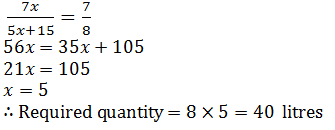NABARD Quantitative Aptitude Practice: 3 - Question 8

In an innings of a cricket match, three players A, B and C socored a total of 361 runs. If the ratio of the number of runs scored by A to that scored by B and also number of runs scored by B to that scored by C be 3 : 2, the number of runs scored by A was

Detailed Solution for NABARD Quantitative Aptitude Practice: 3 - Question 8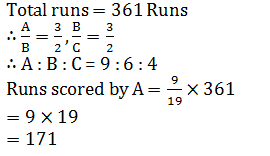NABARD Quantitative Aptitude Practice: 3 - Question 9

A man and a boy received Rs. 800 as wages for 5 days for the work they did together. The man’s efficiency is twice of the boy. What are the daily wages of the boy?

Detailed Solution for NABARD Quantitative Aptitude Practice: 3 - Question 9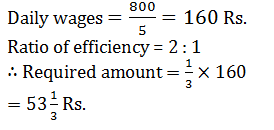NABARD Quantitative Aptitude Practice: 3 - Question 10

In a circular race of 900m length, A and B start with speeds 27 km/hr and 36 km/hr starting at the same time from the same point. When will they meet for the first time at the starting point when running in opposite direction?

Detailed Solution for NABARD Quantitative Aptitude Practice: 3 - Question 10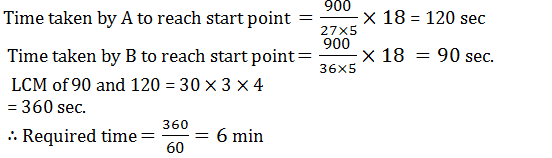NABARD Quantitative Aptitude Practice: 3 - Question 11

Following line graph shows the percentage profit earned by two companies 'A' and 'B' during the period of 2007 to 2013: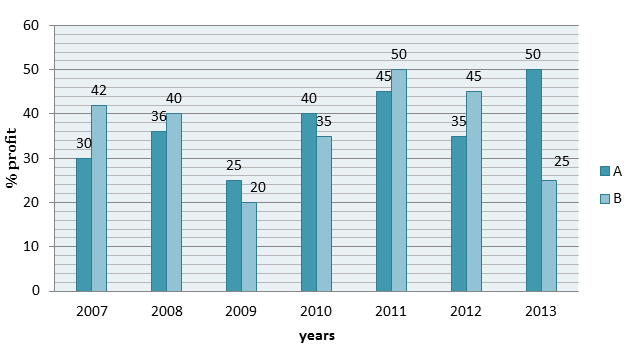Q. If the expenditure of company A and B in year 2008 was 16.5 lakh and 20.8 lakh respectively then what was the income of company A and company B together in year 2008?

Detailed Solution for NABARD Quantitative Aptitude Practice: 3 - Question 11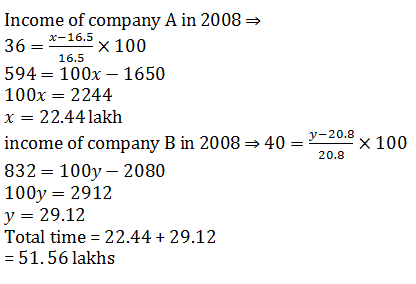NABARD Quantitative Aptitude Practice: 3 - Question 12

Following line graph shows the percentage profit earned by two companies 'A' and 'B' during the period of 2007 to 2013:Q. If the income of company A in 2011 and that of B in 2010 are Rs 24.36 lakh and Rs 18.36 lakh respectively then what is the total expenditure of company A in 2011 and company B in 2010?

Detailed Solution for NABARD Quantitative Aptitude Practice: 3 - Question 12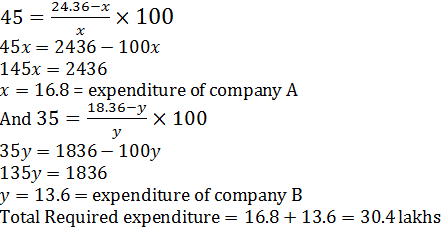NABARD Quantitative Aptitude Practice: 3 - Question 13

Following line graph shows the percentage profit earned by two companies 'A' and 'B' during the period of 2007 to 2013:​Q. If the expenditure of company A in 2009 and income of company B in 2013 are equal then what will be the ratio of income of company A in 2009 and expenditure of company B in 2013?

Detailed Solution for NABARD Quantitative Aptitude Practice: 3 - Question 13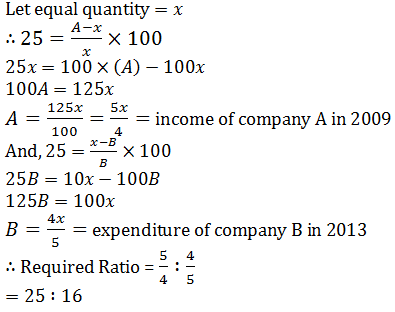NABARD Quantitative Aptitude Practice: 3 - Question 14

Following line graph shows the percentage profit earned by two companies 'A' and 'B' during the period of 2007 to 2013:​Q. If the expenditure of company A in 2012 and company B in 2009 are equal then the income of company A in 2012 is approximately what percentage of income of company B in 2009?

Detailed Solution for NABARD Quantitative Aptitude Practice: 3 - Question 14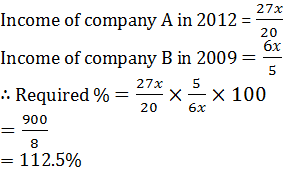NABARD Quantitative Aptitude Practice: 3 - Question 15

Following line graph shows the percentage profit earned by two companies 'A' and 'B' during the period of 2007 to 2013:Q. In the following years in which year the percentage rise/fall percent profit of company B is maximum compare to its previous year?

NABARD Quantitative Aptitude Practice: 3 - Question 16

What should come in place of question mark (?) in the following number series?

3, 14, 39, 84, 155, ?

Detailed Solution for NABARD Quantitative Aptitude Practice: 3 - Question 16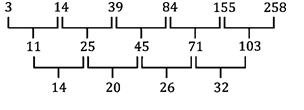NABARD Quantitative Aptitude Practice: 3 - Question 17

What should come in place of question mark (?) in the following number series?

5, 8, 21, 52, 109, ?

Detailed Solution for NABARD Quantitative Aptitude Practice: 3 - Question 17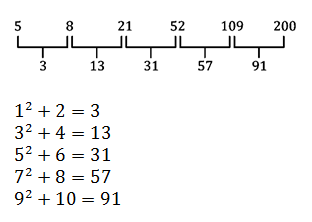NABARD Quantitative Aptitude Practice: 3 - Question 18

What should come in place of question mark (?) in the following number series?

18, 19, 36, 111, 440, ?

Detailed Solution for NABARD Quantitative Aptitude Practice: 3 - Question 18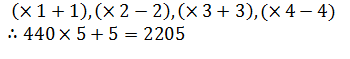NABARD Quantitative Aptitude Practice: 3 - Question 19

What should come in place of question mark (?) in the following number series?

24, 36, 72, 180, 540, ?

Detailed Solution for NABARD Quantitative Aptitude Practice: 3 - Question 19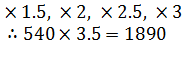NABARD Quantitative Aptitude Practice: 3 - Question 20

What should come in place of question mark (?) in the following number series?

150, 205, 160, 195, 170, ?

Detailed Solution for NABARD Quantitative Aptitude Practice: 3 - Question 20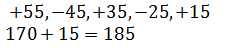NABARD Quantitative Aptitude Practice: 3 - Question 21

Refer to the information given in the graphs below and answer the questions that follow.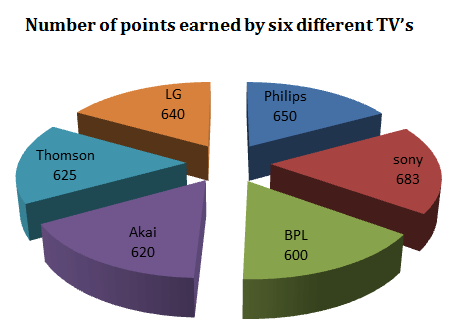(of the total points received assume that the following is the breakup points received due to each of the parameters listed below.)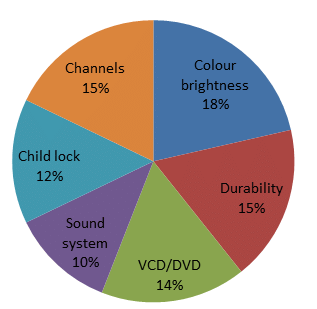Q. The points obtained by Thomson for durability is equal to which of the following?

Detailed Solution for NABARD Quantitative Aptitude Practice: 3 - Question 21

Points obtained by Thomson for durability and channels are equal.

NABARD Quantitative Aptitude Practice: 3 - Question 22

Refer to the information given in the graphs below and answer the questions that follow.(of the total points received assume that the following is the breakup points received due to each of the parameters listed below.)Q. What is total number of the points earned by all the TV’s for channels?

Detailed Solution for NABARD Quantitative Aptitude Practice: 3 - Question 22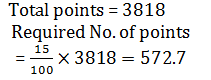NABARD Quantitative Aptitude Practice: 3 - Question 23

Refer to the information given in the graphs below and answer the questions that follow.(of the total points received assume that the following is the breakup points received due to each of the parameters listed below.)Q. What are the average points per TV earned for child lock?

Detailed Solution for NABARD Quantitative Aptitude Practice: 3 - Question 23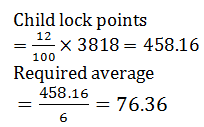NABARD Quantitative Aptitude Practice: 3 - Question 24

Refer to the information given in the graphs below and answer the questions that follow.(of the total points received assume that the following is the breakup points received due to each of the parameters listed below.)Q. The sound system of LG is approximately what per cent of the VCD/DVD of Sony?

Detailed Solution for NABARD Quantitative Aptitude Practice: 3 - Question 24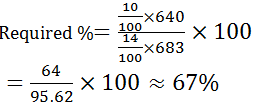NABARD Quantitative Aptitude Practice: 3 - Question 25

Refer to the information given in the graphs below and answer the questions that follow.(of the total points received assume that the following is the breakup points received due to each of the parameters listed below.)Q. The point earned by Philips for VCD/DVD is what percent more/less than the points earned by BPL for colour brightness?

Detailed Solution for NABARD Quantitative Aptitude Practice: 3 - Question 25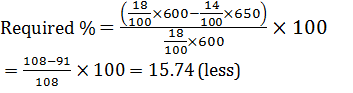NABARD Quantitative Aptitude Practice: 3 - Question 26

What will come in place of the question-mark (?) in the following questions?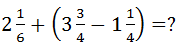Detailed Solution for NABARD Quantitative Aptitude Practice: 3 - Question 26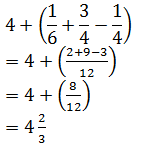NABARD Quantitative Aptitude Practice: 3 - Question 27

What will come in place of the question-mark (?) in the following questions?
Q. 36251 + 43261 = ? + 52310

Detailed Solution for NABARD Quantitative Aptitude Practice: 3 - Question 27

27202

NABARD Quantitative Aptitude Practice: 3 - Question 28

What will come in place of the question-mark (?) in the following questions?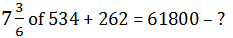Detailed Solution for NABARD Quantitative Aptitude Practice: 3 - Question 28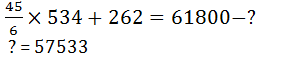NABARD Quantitative Aptitude Practice: 3 - Question 29

What will come in place of the question-mark (?) in the following questions?
72% of 486 – 64% of 261 = ?

Detailed Solution for NABARD Quantitative Aptitude Practice: 3 - Question 29

349.92-167.04=182.88

NABARD Quantitative Aptitude Practice: 3 - Question 30

What will come in place of the question-mark (?) in the following questions?
? ÷ 62 × 12 = 264

Detailed Solution for NABARD Quantitative Aptitude Practice: 3 - Question 30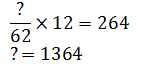## NABARD Assistant Manager Grade B Mock Test Series

1 docs|25 tests
 Use Code STAYHOME200 and get INR 200 additional OFF Use Coupon Code
Information about NABARD Quantitative Aptitude Practice: 3 Page
In this test you can find the Exam questions for NABARD Quantitative Aptitude Practice: 3 solved & explained in the simplest way possible. Besides giving Questions and answers for NABARD Quantitative Aptitude Practice: 3, EduRev gives you an ample number of Online tests for practice

1 docs|25 tests